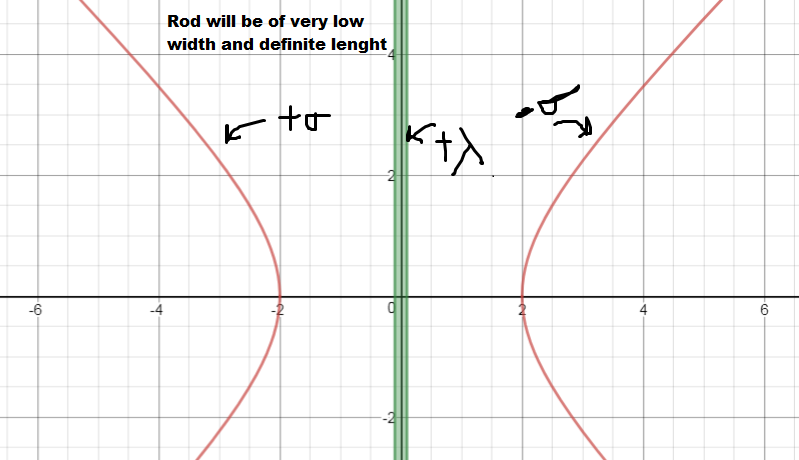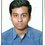# A Doubt on Electrostatics ( Inspired From Steven Sir)

You can check out this one first!.... https://brilliant.org/problems/focused-electric-field/?ref_id=1480760

QUESTION

Now my question is something different, Say we have a rectangular hyperbola, $x^2-y^2=a^2$ of which the right hand part is charged with linear charge density $-\sigma$ and the left one with $+ \sigma$ .

There is a Rod Lying on the y-axis, with mass $M$, Lenght $2L$ and its center is kept at $(0,0)$. It is charged with, say surface charge density, $+\lambda$.

Check out the image.This is it.

So We need to find the acceleration of the rod, as soon as we release it.

SOLUTION AS DONE BY ME (Not sure if correct).

If we draw the interaction between one element from the hyperbola to the rod, we can see that, The net force acting is in the X-direction and the Y- direction cuts off.

And The force from two opposite charged hyperbola on the rod will add up and will be like $dF_{x}=2 \times dF \sin \theta$.

$\implies dF_{x}= \dfrac{2 \times dq_{1} \times dq_{2} \sin \theta }{(x^2+(y-l)^2)}$

Here (x,y) is the position of the charges on the hyperbola. and $l$ is the distance from the center of the rod to the element. and $\theta$ is the acute angle made by the rod with the line joining the element of rod and hyperbola.

$\implies dF_{x}= \dfrac{2 \times dq_{1} \times dq_{2} x }{(x^2+(y-l)^2)^{\tiny{\dfrac{3}{2}}}}$

We know $dq_{1} = \sigma dy \sqrt{1+(\dfrac{dx}{dy})^2}$ whereas dq = $\lambda dl$ ,

now putting these in the equation the force of interaction on the Rod comes out to be,

$\boxed{F=\displaystyle{\int_{-\infty}^{\infty} \int_{-L}^{+L} \dfrac{2 \lambda \sigma \sqrt{2y^2+a^2} dl dy}{(y^2+(y-l)^2+a^2)^{1.5}}}}$

## $\boxed{A=\displaystyle{\int_{-\infty}^{\infty} \int_{-L}^{+L} \dfrac{2 \lambda \sigma \sqrt{2y^2+a^2} dl dy}{(y^2+(y-l)^2+a^2)^{1.5} \times M}}}$

I want you guys to verify if I am correct at every places and our expression comes same.

Question is original.

Please do the verification fast! I want to make a question on this and post it! And if you can do the integral, please tell me what comes.

Thanks again!Note by Md Zuhair
3 years, 4 months ago

This discussion board is a place to discuss our Daily Challenges and the math and science related to those challenges. Explanations are more than just a solution — they should explain the steps and thinking strategies that you used to obtain the solution. Comments should further the discussion of math and science.

When posting on Brilliant:

• Use the emojis to react to an explanation, whether you're congratulating a job well done , or just really confused .
• Ask specific questions about the challenge or the steps in somebody's explanation. Well-posed questions can add a lot to the discussion, but posting "I don't understand!" doesn't help anyone.
• Try to contribute something new to the discussion, whether it is an extension, generalization or other idea related to the challenge.

MarkdownAppears as
*italics* or _italics_ italics
**bold** or __bold__ bold
- bulleted- list
• bulleted
• list
1. numbered2. list
1. numbered
2. list
Note: you must add a full line of space before and after lists for them to show up correctly
paragraph 1paragraph 2

paragraph 1

paragraph 2

[example link](https://brilliant.org)example link
> This is a quote
This is a quote
    # I indented these lines
# 4 spaces, and now they show
# up as a code block.

print "hello world"
# I indented these lines
# 4 spaces, and now they show
# up as a code block.

print "hello world"
MathAppears as
Remember to wrap math in $$ ... $$ or $ ... $ to ensure proper formatting.
2 \times 3 $2 \times 3$
2^{34} $2^{34}$
a_{i-1} $a_{i-1}$
\frac{2}{3} $\frac{2}{3}$
\sqrt{2} $\sqrt{2}$
\sum_{i=1}^3 $\sum_{i=1}^3$
\sin \theta $\sin \theta$
\boxed{123} $\boxed{123}$

Sort by:

- 3 years, 4 months ago

Hello. The approach looks right to me. I have two comments:

1) Under the radical in your numerator, doesn't it need to be $\large{\frac{2 y^2 + a^2}{y^2 + a^2}}$?
2) I'm not sure how to evaluate the integral formally, but it is no trouble to approximate numerically

- 3 years, 4 months ago

Never mind. When you bring in the x, it cancels the denominator. Looks good

- 3 years, 4 months ago

Ya sir, I did that only, Okay, sir, Would you help me evaluate it by taking

$M=1 kg$ ,$L=2m$ and $a=2m$ , $\lambda = 5 C/m$ and $\sigma= 10 C/m$

Then i will delete the doubt and post an question!

- 3 years, 4 months ago

Well, I spoke a little too soon when I said "no trouble". It's easy enough to write code to evaluate it. But then I would have to vary parameters (integration step size, integration window, etc.) and make sure that the convergence is good. I need to go to bed now, but I'd say you can give the numerical integration a shot and post it.

- 3 years, 4 months ago

Okay sir! Will Try it! And which language do you use to do calculus in computer?

- 3 years, 4 months ago

Sounds good. I use Python. It's not as simple as calling an "integrate" function, but you can easily do a Riemann sum (or a double Riemann sum).

- 3 years, 4 months ago

Oh nice, I see... Sir according to https://www.integral-calculator.com/ , I got 410.372632 . Should I try Posting it?

- 3 years, 4 months ago

If you are confident in the answer, go ahead and post it. I'll try it tomorrow. It's a neat problem

- 3 years, 4 months ago

Okay sir! Sure, Lets post, if reports come, I will delete it and correct it. Thanks for Helping me! I guess in US, it night! So goodnight sir! Here , In India, Its 1:33 pm :)

- 3 years, 4 months ago

@Md Zuhair Hiya!! I see that you are so much advanced in physics.....That's OSM..!! Do you mind if I ask that where do u learn it?? I am referring to Resnick Halliday, and have only finished the first 7-8 chapters......(Mechanics excluding rotation).....But I need to improve my skills.....any tips??

- 3 years, 4 months ago

Arre! Waise nai hai. Ok, So I do learn from Books, Youtube channels and Brilliant (90%) and learn many new concepts on solving problems. It clears out my concepts, Actually, Do not ask, Where did you learn, Ask From where not to learn, You should try learning from everywhere. Kuch zyada hi bol diya, Lagta Hai. Ok.. So Resnick Halliday is good, and try learning Rotation fast, it still is difficult to me in some respects!

Thanks :D!

- 3 years, 4 months ago

Ohhh I see.....zyaada nahi bola hai.....!! I am more into Maths than any other subject aur ab mujhe Phy and Chem bhi improve karni hain....( I have not even started the NCERT of Chem....!! well except that atomic structure waala chapter!! )....Ok....then I'll first finish off Resnick!!
Thanks to u man!!

- 3 years, 4 months ago

Arre .. no thanks. Best of luck

- 3 years, 4 months ago

I posted some example code under the one with the tension and the bob.

https://brilliant.org/problems/breaking-string/

- 3 years, 4 months ago

Okay! Will check it out

- 3 years, 4 months ago

@Chew-Seong Cheong , Sir, Can you make this look prettier?

https://brilliant.org/problems/complex-harmonic-motion/?ref_id=1484330

- 3 years, 4 months ago

Bro !! Abhi bhi online!! Sorry I won't try ur problem....

- 3 years, 4 months ago